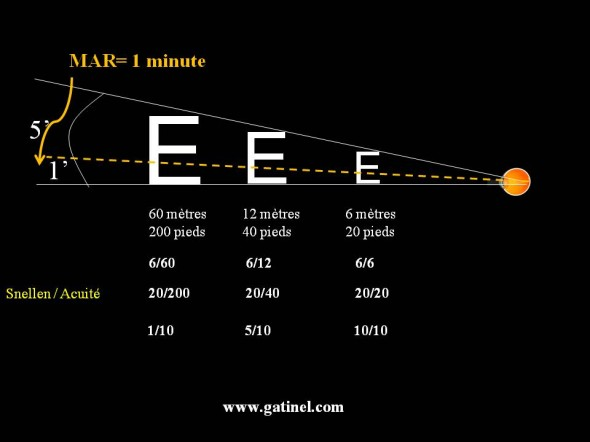+ +

# MAR: minimum angle of resolution

The angle MAR or AMR (minimum angle of resolution) is the Visual angle that must under tender two point sources to be resolved on the retina of the eye - MAR is an acronym for "Minimum Angle of Resolution". The calculation of the MAR depends on parameters such as the diameter of the pupil, the presence of a defective vision (myopia, hypermetropia, etc.) and refers to the notion ofVisual acuity  resolution, that is to say the ability to separate two points; find out the MAR angle is a way to quantify the resolving power of the eye.

The minimum angle of resolution (MAR) judged as 'normal' is by convention of 1 minute of arc, or 1 / 60 ° of level.

Visual acuity is equal to the inverse of the MAR angle: an angle of minimum resolution of 1 min corresponds to an acuity of 1/1 or 10/10 (acuity decimal, expressed in tenths).

A MAR of 1 arc minutes corresponds to a Visual acuity of 10/10.

The power of theoretical resolution of the foveolaire retina is actually 20/10 - either half a minute of arc or 30 seconds of arc, but in practice we're holding 10/10 as the threshold for defining a Visual acuity 'normal').

In ophthalmology, estimated the MAR through the measurement of Visual acuity, which is performed by asking the patient to identify a series of letter of decreasing size projected on a screen. One Visual acuity 10/10 is such that a letter E 6 metres can be recognized if she measures 8 mm in height.  In those circumstances, the letter E is part of an angle of 5 arc minutes, and each bar of the E under tends an angle of 1 minute of arc (the E is made up of 5 horizontal bars: 3 black, and two intertwined white). To see the E, each bar is "separate" on the retina with an angle of 1 minute of arc.When theVisual acuity is 10/10, minimum resolution angle (MAR) is 1 minute of arc. If the angle double (2 minutes of arc), Visual acuity drops by half, as equal to the inverse of the MAR angle, it is worth either 1/2 0.5 so 5/10. When Visual acuity is 9/10, the MAR is 1.11 minute of arc (10/9). A 5/10, this angle is 2 minutes of arc, and 1/10 is a 10/1 = 10 minutes of bow MAR. The gap between each decimal acuity line does not correspond to a variation of constant amplitude for the Angel of Mar. More decimal Visual acuity is high, and the gain of sharpness for the addition of a line represents a slight reduction of the Mar.

This geometric progression suits bad statistical calculations such as average arithmetic. That is why we prefer to choose the logarithm to the base 10 (Log) of the angle of minimum resolution (MAR): the LogMAR.